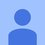# My teacher gave me this tricky problem

the number of 6-digit numbers of the form ababab (in base 10) each of which is a product of exactly 6 distinct primes is (1) 8 (2) 10 (3)13 (4) 15 please reply friends.4 years, 6 months ago

This discussion board is a place to discuss our Daily Challenges and the math and science related to those challenges. Explanations are more than just a solution — they should explain the steps and thinking strategies that you used to obtain the solution. Comments should further the discussion of math and science.

When posting on Brilliant:

• Use the emojis to react to an explanation, whether you're congratulating a job well done , or just really confused .
• Ask specific questions about the challenge or the steps in somebody's explanation. Well-posed questions can add a lot to the discussion, but posting "I don't understand!" doesn't help anyone.
• Try to contribute something new to the discussion, whether it is an extension, generalization or other idea related to the challenge.

MarkdownAppears as
*italics* or _italics_ italics
**bold** or __bold__ bold
- bulleted- list
• bulleted
• list
1. numbered2. list
1. numbered
2. list
Note: you must add a full line of space before and after lists for them to show up correctly
paragraph 1paragraph 2

paragraph 1

paragraph 2

[example link](https://brilliant.org)example link
> This is a quote
This is a quote
    # I indented these lines
# 4 spaces, and now they show
# up as a code block.

print "hello world"
# I indented these lines
# 4 spaces, and now they show
# up as a code block.

print "hello world"
MathAppears as
Remember to wrap math in $$ ... $$ or $ ... $ to ensure proper formatting.
2 \times 3 $2 \times 3$
2^{34} $2^{34}$
a_{i-1} $a_{i-1}$
\frac{2}{3} $\frac{2}{3}$
\sqrt{2} $\sqrt{2}$
\sum_{i=1}^3 $\sum_{i=1}^3$
\sin \theta $\sin \theta$
\boxed{123} $\boxed{123}$

Sort by:

The $6-digit$ $number$ is in the form of $\color{#D61F06}{\overline{ababab}}$.

Therefore ,

$\Rightarrow \color{#D61F06}{\overline{ababab}}$

$\Rightarrow 10^{5}a+10^{4}b+10^{3}a+10^{2}b+10a+b$

$\Rightarrow 101010a+10101b$

$\Rightarrow 10101(10a+b)$

$\Rightarrow 3×7×13×37×(10a+b)$

Now by observing the above line , we can say that $(10a+b)$ should have two prime factors other than $3,7,13$ and $37$.

Also when we see $(10a+b)$ , we find that it is just a two digit number.

Now finding the number of numbers from $10-100$ having two prime factors.

$Case I$:

Taking $2$ as one of the prime factors , we can say the upper limit as $2×50=100$ which means that the other prime number should be $2 as we discussed earlier that the number should be of $2$ digits.

Therefore , number of prime numbers $2 is $14$ but we don't have to count $3,7,13$ and $37$.Thus , there are $\huge 10$ such numbers.

$Case II$:

Now taking $5$ as one of the prime factors , the upper limit is $5×20=100$. By this we can say that the another prime number should be $5.Now there are $5$ prime numbers $5 but $7$ and $13$ are not to be considered. Therefore , there are $\huge 3$ such numbers.

Only these are the possibilities.

Therefore , Answer=$\huge \boxed{13}$

- 4 years, 6 months ago

Thanks Akshat for your amazing solution. You have proved the title to be wrong. I wish I could upvote your solution thousand times. And thank you Mohit for your answer.

- 4 years, 6 months ago

^_^

- 4 years, 6 months ago

Nice!

Staff - 4 years, 6 months ago

$\huge Thanks!!$

- 4 years, 6 months ago

Yes , its $13$.

- 4 years, 6 months ago

- 4 years, 6 months ago

you may be correct since i don't know the correct answer so please write the solution and thank you for your instant reply. i was not able to answer this question so i made a note.

- 4 years, 6 months ago

I think that you'll find my solution useful ^_^

- 4 years, 6 months ago